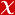## The Diophantine equation (x+1)(k) + (x+2)(k) + ... plus (lx)(k) = y(n) revisted

PUBLICATIONES MATHEMATICAE-DEBRECEN, vol.96, no.1-2, pp.111-120, 2020 (SCI-Expanded)• Publication Type: Article / Article
• Volume: 96 Issue: 1-2
• Publication Date: 2020
• Doi Number: 10.5486/pmd.2020.8604
• Journal Name: PUBLICATIONES MATHEMATICAE-DEBRECEN
• Journal Indexes: Science Citation Index Expanded (SCI-EXPANDED), Scopus, MathSciNet, zbMATH, DIALNET
• Page Numbers: pp.111-120
• Keywords: Bernoulli polynomials, high degree equations, PERFECT POWERS, SUMS
• Bursa Uludag University Affiliated: Yes

#### Abstract

Let k,l >= 2 be fixed integers, and C be an effectively computable constant depending only on k and l. In this paper, we prove that all solutions of the equation (x + 1)(k) + (x + 2)(k) + ... + (lx)(k) = y(n) in integers x, y,n with x, y >= 1, n >= 2, k not equal 3 and l 1 (mod 2) satisfy max{x, y, n} < C. The case when is even has already been completed by the second author (see ).